# CBSE Question Paper 2006 class 12 Chemistry## myCBSEguide App

Complete Guide for CBSE Students

NCERT Solutions, NCERT Exemplars, Revison Notes, Free Videos, CBSE Papers, MCQ Tests & more.

CBSE Question Paper 2006 class 12 Chemistry conducted by Central Board of Secondary Education, New Delhi in the month of March 2006. CBSE previous year question papers with the solution are available in the myCBSEguide mobile app and website. The Best CBSE App for students and teachers is myCBSEguide which provides complete study material and practice papers to CBSE schools in India and abroad.

CBSE Question Paper 2006 class 12 Chemistry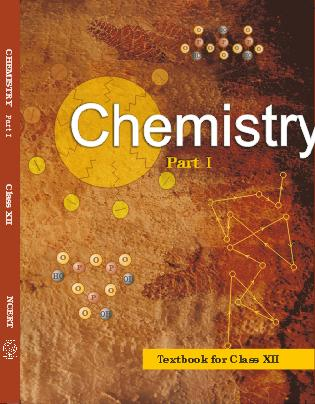## Class 12 Chemistry list of chapters

1. The Solid State
2. Solutions
3. Electrochemistry
4. Chemical Kinetics
5. Surface Chemistry
6. General Principles and Processes of Isolation of Elements
7. The p-Block Elements
8. The d and f Block Elements
9. Coordination Compounds
10. Haloalkanes and Haloarenes
11. Alcohols, Phenols and Ethers
12. Aldehydes, Ketones and Carboxylic Acids
13. Amines
14. Biomolecules
15. Polymers
16. Chemistry in Everyday life

## CBSE Question Paper 2006 class 12 Chemistry

### General Instructions

1. All questions are compulsory.
2. Marks for each question are indicated against it.
3. Question numbers 1 to 5 are very short-answer questions, carrying 1 mark each. Answer these in one word or about one sentence each.
4. Question numbers 6 to 12 are short-answer questions, carrying 2 marks each. Answer these in about 30 words each.
5. Question numbers 13 to 24 are short-answer questions of 3 marks each. Answer these in about 40 words each.
6. Question numbers 25 to 27 are long-answer questions of 5 marks each. Answer these in about 70 words each.
7. Use Log Tables, if necessary Use of calculators is not permitted.

1. Name the crystal which lowers the density of an ionic crystal. 

2. Of 0.1 molal solutions of glucose and sodium chloride respectively, which one will have a higher boiling point? 

3. What is meant by elementary step in a reaction? 

4. Draw the structural formula of hex-2-en-4-y-noic acid. 

5. Why are aqueous solutions of amines basic in nature? 

6. How does the principal quantum number (say n = 4) of an energy level of electrons in an atom limit the maximum number of electrons that can be accommodated at that level? 

7. Give an example of a spontaneous process which is endothermic. What makes it spontaneous? 

8. What happens when

i.  Chlorine is passed through a hot concentrated solution of an alkali like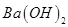?

ii.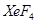undergoes hydrolysis?

Write the chemical equation in each case. 

9. What is meant by ‘lanthanoid contraction’? State one use each of lanthanoid metals and their oxides. 

10. Draw three dimensional representations of 2-butanol which are of R and S type. 

11. How are the following conversions carried out?

i.  Benzyl chloride to benzyl alcohol

ii.  Ethyl magnesium bromide to propan-1-ol 

12. Write the names of monomers used for getting the polymers PVC and PMMA. State one use for each of these polymers. 

Or

Giving one example for each differentiate between thermosetting and thermo plastic polymers.

13. i.  Explain why the bond order of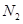is greater than that of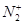while the bond order of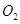is less than that of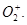ii.  How does the bond order concept support helium molecules being monatomic and oxygen molecules being paramagnetic? 

14. Explain the following with suitable examples: 

a.  An n-type semiconductor

b.  Piezoelectric effect

Or

a.  With reference to a crystal structure, what is meant by coordination number?

b.  What is the coordination number of atoms

i.  in a cubic close packed structure,

ii.  in a body-centred cubic structure?

15. At 300 K 36 g of glucose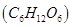present per litre in its aqueous solution has an osmotic pressure of 4.98 bar. If the osmotic pressure of another solution of glucose is 1.52 bar at the same temperature, what would be its concentration? 

16. In a fuel cell (a device for producing electricity directly from a chemical reaction), methanol is used as a fuel and oxygen gas is used as an oxidiser. The reaction is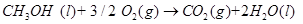Calculate the standard Gibbs energy change for the reaction that can be converted into electrical work.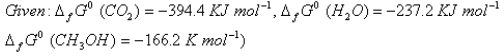17. At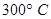the thermal dissociation of HI is found to be 20%. What will be the equilibrium concentrations of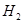and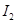in the system at this temperature if the equilibrium concentration of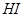in it be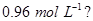18. Write three distinct differences between physical adsorption and chemisorptions. 

19. Assign reasons for the following observations 

i.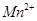compounds are more stable than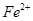compounds towards oxidation to their +3 state.

ii.  Interstitial compounds are well known for transition elements.

iii.  An aqueous solution of potassium chromate is yellow but changes its colour on decreasing the pH of the solution.

20. Write the name (IUPAC norm) and draw the possible optical isomers of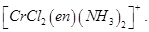. 

21. Complete the following nuclear reaction symbolizations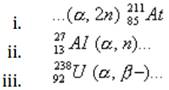(Note: you can put ‘X’ if the exact unknown symbol is not known)

22. Describe the following giving a chemical equation for each: 

i.  Cannizzaro’s reaction

ii.  Transesterification

iii.  Hofmann bromamide reaction

23. Answer the following giving reaction conditions and a complete chemical equation in each case: 

i.  How would you prepare ethyl amine from acetaldehyde?

ii.  How can you get benzonitrile from aniline?

iii.  How is ethyl isonitrile obtained from ethyl amine?

24. Describe the following giving a suitable example in each case: 

i.  Antioxidants

ii.  Mordant dyes

iii.  Hybrid propellants

25. a.  Define the terms specific conductance and molar conductivity for solutions of electrolytes.

b.  Write the cell formulation and calculate the standard cell potential of the galvanic cell in operation of which the following reaction takes place: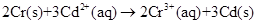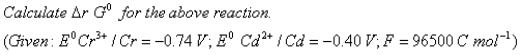[2, 3]

Or

a.  Explain with an example how weak and strong electrolytes can be distinguished.

b.  In the button cell used in watches the following reaction occurs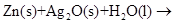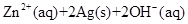Determine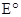for the cell and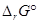for the reaction.

(Given: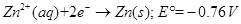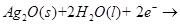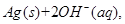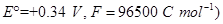26. a.  How would you account for the following observations:

i.  The tendency for catenation decreases as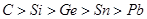in Group 14 of the periodic table.

ii.  The pentavalent Bismuth is a strong oxidizing agent.

iii.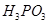is a diprotic acid.

b.  Draw the structural formulae of the following molecules:

i.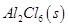ii.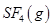[3, 2]

Or

a.  Explain the following observations giving an appropriate reason in each case:

i.  The +1 oxidation state of thallium is more stable than its +3 oxidation state.

ii.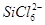is not known to exist whereas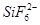exists.

iii.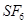is inert towards hydrolysis.

b.  Draw the structural formulae of the following molecules:

i.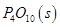ii.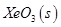27. a.  What are the light and the dark stages in photosynthesis in green plants? Give the basic equation of photosynthesis.

b. i.  Which forces are responsible for the stability of ά-helix?

ii.  What is a denatured protein? [3, 2]

Or

a.  What are reducing and non-reducing sugars? What is the structural feature characterizing reducing sugars? What is invert sugar?

b.  Define enzymes. What is the most important reason for their specificity in action?

These are questions only. To view and download complete question paper with solution install myCBSEguide App from google play store or login to our student dashboard.

## Chemistry Question Paper 2006

Download class 12 Chemistry question paper with solution from best CBSE App the myCBSEguide. CBSE class 12 Chemistry question paper 2006 in PDF format with solution will help you to understand the latest question paper pattern and marking scheme of the CBSE board examination. You will get to know the difficulty level of the question paper. CBSE question papers 2006 for class 12 Chemistry have 27 questions with solution.

## Previous Year Question Paper for class 12 in PDF

CBSE question papers 2018, 2017, 2016, 2015, 2014, 2013, 2012, 2011, 2010, 209, 2008, 2007, 2006, 2005 and so on for all the subjects are available under this download link. Practicing real question paper certainly helps students to get confidence and improve performance in weak areas.

To download CBSE Question Paper 2006 class 12 Accountancy, Chemistry, Physics, History, Political Science, Economics, Geography, Computer Science, Home Science, Accountancy, Business Studies and Home Science; do check myCBSEguide app or website. myCBSEguide provides sample papers with solution, test papers for chapter-wise practice, NCERT solutions, NCERT Exemplar solutions, quick revision notes for ready reference, CBSE guess papers and CBSE important question papers. Sample Paper all are made available through the best app for CBSE students and myCBSEguide website.## Test Generator

Create Papers with your Name & Logo